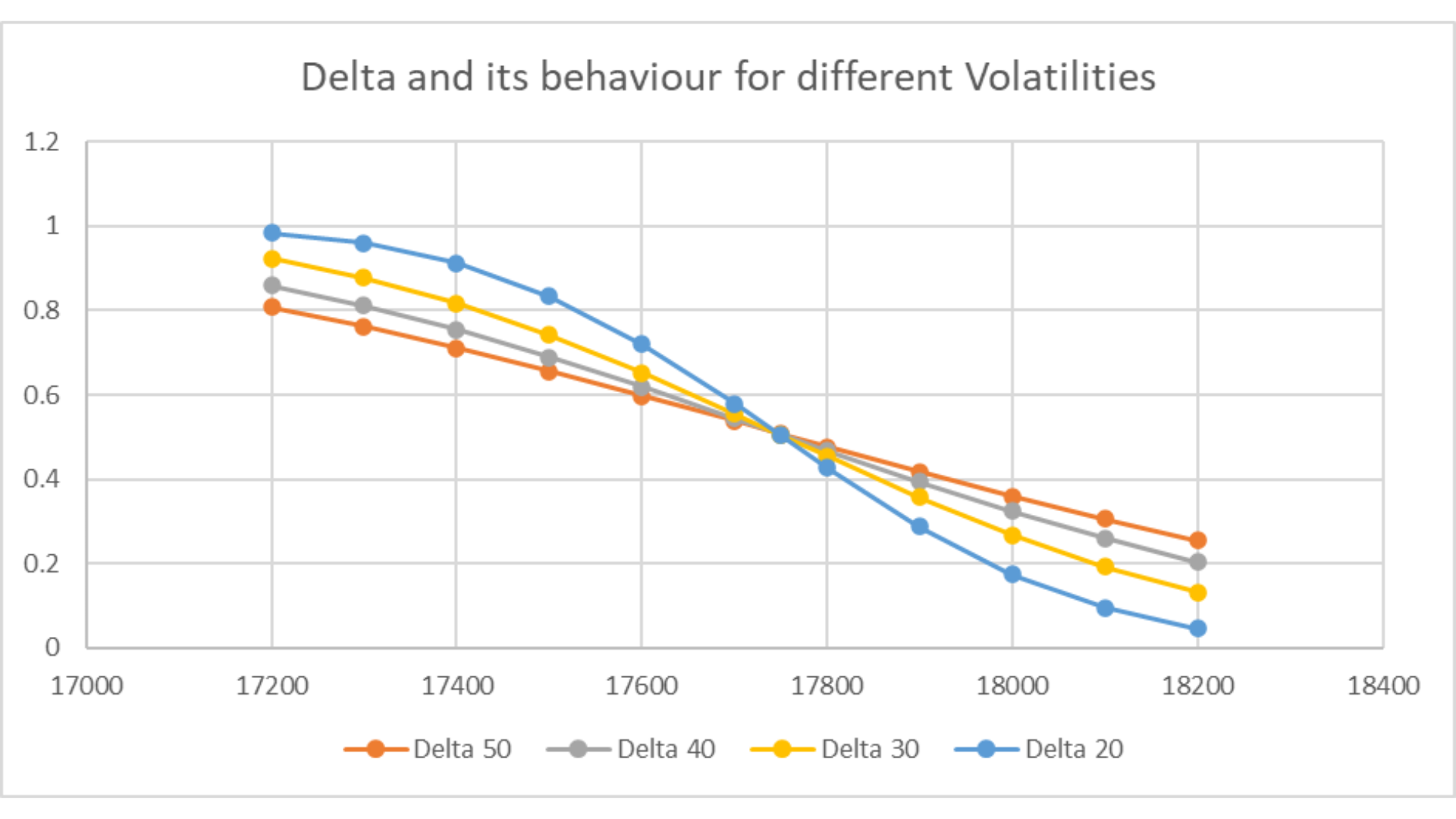VannaVanna

# Vanna

Vanna is second order greek, it is the ratio of change in vega to the change in underlying. Alternatively, it is the ratio of change in delta to the change in volatility. How do we arrive at it? Let’s look at the definition:So, essentially Vanna, is the behaviour of Delta, when volatility jumps or recedes. As volatility rises, the time value of the option goes up and this causes the delta of out-of-the-money options to increase and the delta of in-the-money options to decrease. The Vanna is negative for ITM options, while positive for OTM options.The Delta 20 curve indicates the value of Option Greek, Delta across strikes of Nifty call options, with Days to expiry (DTE) = 2, volatility = 20%. It is evident from the above chart that as volatility of Nifty or any F&O stock increases from 20% to 30% to 50%, the Delta curve tends to flatten out and move towards the ATM option delta of 0.5.

This tendency of different deltas to head towards the ATM delta, is due to “Kuch Bhi Ho Sakta Hain Syndrome”, i.e. as volatility increases the ITM options have the tendency to become OTM and vice versa. Hence all strikes’ delta move closer to 50% or 0.5. So, Vanna is 0 for ATM options. Vanna is negative for ITM options and positive for OTM options.

### What are the effects of option Greek, Vanna?

Vanna is a second-order option Greek that measures the sensitivity of the delta of an option to changes in the implied volatility of the underlying asset. In other words, Vanna measures the rate of change of delta with respect to changes in volatility. Since Vanna measures the sensitivity of delta to changes in implied volatility, changes in Vanna can lead to changes in the option's delta. Specifically, if Vanna is positive, an increase in volatility will lead to an increase in the option's delta, while a decrease in volatility will lead to a decrease in the option's delta. Conversely, if Vanna is negative, changes in volatility will have the opposite effect on delta.

### What are the disadvantages of Vanna?

1. Vanna is a second-order Greek, which means that it is more complex to calculate than the first-order Greeks (such as delta, gamma, and theta). As a result, it can be more difficult to understand and use for some traders, especially those who are new to options trading.

2. Vanna is highly sensitive to changes in implied volatility, which can make it difficult to use in rapidly changing market conditions. For example, in a volatile market, Vanna can change quickly, making it challenging to manage risk effectively.

3. Vanna is based on the Black-Scholes pricing model, which assumes certain conditions that may not always hold true in the real world. For example, the model assumes that the underlying asset's volatility is constant over time, which is often not the case in reality. Black-Scholes model also assumes normal distribution.

### What impact does volatility spike on option deltas, of different moneyness?

Option delta remains at 0.5 for the ATM call options and -0.5 for the ATM put options. The impact of volatility for ATM options is negligible on option delta. However, a sharp surge in volatility suppresses the ITM call option delta toward 0.5 and causes the OTM call option delta to transition gradually towards the 0.5 mark. The high volatility regime causes the “Kuch bhi ho sakta hain” syndrome, i.e. “Anything can happen”.